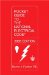# Example No. D3 Store Building

A store 50 ft. by 60 ft., or 3000 sq. ft., has 30 ft. of show window. There are a total of 80 duplex receptacles. The service is 120/240-volt, single-phase 3-wire service. Actual connected lighting load, 8500 volt-amperes.

 Calculated Load (See 220.40) Noncontinuous Loads Receptacle Load (see 220.44) 80 receptacles at 180 VA 14,400 volt-amperes 10,000 VA at 100% 10,000 volt-amperes 14,400 VA - 10,000 VA = 4400 VA at 50% 2200 volt-amperes Subtotal 12,200 volt-amperes Continuous Loads General Lighting[*] 3000 sq. ft. at 3 volt-amperes per sq. ft. 9000 volt-amperes Show Window Lighting Load 30 ft. at 200 volt-amperes per foot 6000 volt-amperes Outside sign circuit 1200 volt- amperes [see 220.14(F)] 1200 volt-amperes Subtotal 16,200 volt-amperes Subtotal from noncontinuous 12,200 volt-amperes Subtotal noncontinuous loads plus continuous loads 28,400 volt-amperes

[*] In the example, 125 percent of the actual connected lighting load (8500 VA x 1.25 = 10,625 VA) is less than 125 percent of the load from Table 220.12, so the minimum lighting load from Table 220.12 is used in the calculation. Had the actual lighting load been greater than the value calculated from Table 220.12, 125 percent of the actual connected lighting load would have been used.

Minimum Number of Branch Circuits Required

General Lighting: Branch circuits need only be installed to supply the actual connected load [see 221.11(B)].

 8500 VA x 1.25 = 10,625 volt-amperes 10,625 volt-amperes ÷ 240 volts = 44 amperes for 3-wire, 120/240 V

The lighting load would be permitted to be served by 2-wire or 3-wire, 15- or 20-ampere circuits with combined capacity equal to 44 amperes or greater for 3-wire circuits or 88 amperes or greater for 2-wire circuits. The feeder capacity as well as the number of branch-circuit positions available for lighting circuits in the panelboard must reflect the full calculated load of 9000 VA x 1.25 = 11,250 volt-amperes.

Show Window

 6,000 VA x 1.25 = 7500 VA 7500 volt-amperes ÷ 240 volts = 31 amperes for 3-wire, 120/240V

The show window lighting is permitted to be served by 2-wire or 3-wire circuits with a capacity equal to 31 amperes or greater for 3-wire circuits or 62 amperes or greater for 2-wire circuits.

Receptacles required by 210.62 are assumed to be included in the receptacle load above if these receptacles do not supply the show window lighting load.

Receptacles

 Receptacle Load: 14,400 volt-amperes ÷ 240 volts = 60 amperes for 3-wire, 120/240 V

The receptacle load would be permitted to be served by 2-wire or 3-wire circuits with a capacity equal to 60 amperes or greater for 3-wire circuits or 120 amperes or greater for 2-wire circuits.

Minimum Size Feeder (or Service) Overcurrent Protection [See 215.3 or Section 230.90]

 Subtotal noncontinuous loads 12,200 volt-amperes Subtotal continuous load @ 125% 16,200 VA x 1.25 20,250 volt-amperes Total 32,450 volt-amperes 32,450 VA ÷ 240 volts = 135 amperes

The next higher standard size is 150 amperes (See 240.6).

Minimum Size Feeder (or Service Conductors) Required [See 215.2, 230.42(A)]

For 120/240-volt, 3-wire system: 32,450 volt-amperes ÷ 240 volts = 135 amperes.

Service or feeder conductor is 1/0 Cu per 215.3 and Table 310.16 (with 75°C terminations).Pocket Guide to the National Electrical Code(R), 2005 Edition (8th Edition)
ISBN: 0131480014
EAN: 2147483647
Year: 2004
Pages: 120